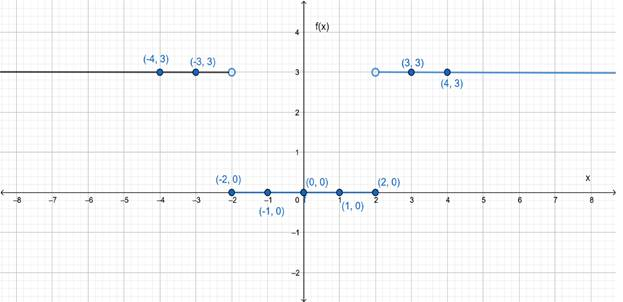# The graph of the given function.### Precalculus: Mathematics for Calcu...

6th Edition
Stewart + 5 others
Publisher: Cengage Learning
ISBN: 9780840068071### Precalculus: Mathematics for Calcu...

6th Edition
Stewart + 5 others
Publisher: Cengage Learning
ISBN: 9780840068071

#### Solutions

Chapter 2.2, Problem 43E
To determine

## To sketch : The graph of the given function.

Expert Solution

### Explanation of Solution

Given information :

f(x)={0     if  |x|23     if  |x|>2

Graph :

|x|22x2

|x|>22>x>2

 x −4 −3 −2 −1 0 1 2 3 4 f(x) 3 3 0 0 0 0 0 3 3This is the graph of the given piecewise defined equation.

Interpretation : The part of the graph to the left of x=2 coincides with the graph of f(x)=3 and the part of the graph to the right of x=2 coincides with the graph of f(x)=3 . The open dot at (2,3) and (2,3) indicates that these points are excluded from the graph. The part of the graph between x=2 and x=2 coincides with f(x)=0 .

### Have a homework question?

Subscribe to bartleby learn! Ask subject matter experts 30 homework questions each month. Plus, you’ll have access to millions of step-by-step textbook answers!